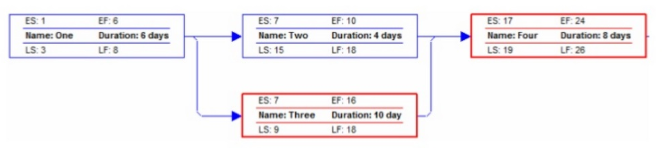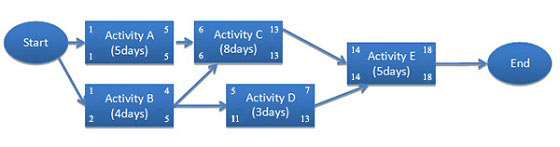Project’s goals can become heavyweights if we don’t have the flexibility to execute intuitive changes. We need the freedom to make choices.  Project Float and Free Float analysis are one of the sources to make choices in the project. We use term float to express flexibility.  This flexibility can be at the project level or the activity level.

During my coaching sessions, I observe a lot of confusion about different types of floats which can exist in a schedule network diagram. Many PMP® aspirants consider total float and free float the same. In this blog, I am drilling this down so that all the confusions go off. And for it, let’s first discuss a few points/definitions:

• During a schedule network diagram – activity can be either predecessor or successor activity corresponding to the dependent activity we refer to
• Predecessor – An activity that logically comes before a dependent activity in a schedule
• Successor – A dependent activity that logically comes after another activity on a schedule.
1. Critical Path – The critical path is the sequence of activities that represents the longest path through a project. It determines the shortest possible project duration
• All activities on the critical path are called critical path activities
2. Schedule Network Diagram – is a graphical representation of the logical relationships. Also referred to as dependencies, among the project schedule activities
3. PDM (Precedence Diagramming Method) – A technique used for constructing a schedule model in which activities are represented by nodes. These are graphically linked by one or more logical relationships to show the sequence in which the activities are to be performed

Here, I am not going into details of above and details of how to create a network diagram, ES, EF, and LS& LF for activities.
Now let’s get back to float. There are 2 types of floats which can exist i.e.

• Total Float
• Free Float

Let’s see what these floats are all about

This is how PMBOK® Guide Sixth Edition defines Total Float

“The amount of time that a schedule activity can be delayed or extended from its early start date without delaying the project ﬁnish date or violating a schedule constraint.”

So this is talking about flexibility we have at the project level. Now in a network diagram, we can have many paths to reach the end. So this float talks about flexibility one path has as compared to others.

Now, the question is how is total float calculated?

Simple formula to calculate total float or slack is our usual formula i.e. LS (Late Start) – ES (Early Start) or LF (Late, Finish) – EF (Early Finish)

Let’s make it simple further to see

How is float time calculated in critical path analysis? And what is total float in critical path method?

The total float and slack are synonymous.

The total float is the difference between project completion date and the total duration of critical path activities.

In other words, you have a project to finish in 25 days. You calculated critical path activities on the schedule network diagram will take 22 days. So you have a project float of +3 days. Here you can see, a float can be a positive or negative number.

We will discuss negative float later in this post.

In summary,

Find the second longest sequence of activities in the network diagram. Subtract its total duration from the duration of the critical path sequence. The difference between the two duration will give you the float for each activity in the second sequence.

I see another confusion here – is Float same as lag? The reason for this confusion lies in the concept of ability to delay an activity. Lag is a delay in the successor activity. Total Float is the amount of time by which you can delay an activity from its early start date without delaying the project finish date.

Then what is the difference between lag and total float?

Both have a different purpose when we look at it from a realistic project management scenario:

Lag is used to put delay deliberately to meet some already available dependency or pre-requisite.  Like you cannot start work of putting wallpaper until the final coat of paint gets done and dried. In this case, you need to delay the wallpaper work by few days.

You can see it is like dependency or necessity.

But, the float is the flexibility we have and helps us to make the desired choices.  It’s up to the project manager if he/she wants to use it or not. e.g., in a project, some path is having six days of float. The project manager uses it to make the best possible decision for the project.

Project Manager may choose to finish an activity on that path by six days in advance. And, then put the resource somewhere else. Or, he/she may first use the resource somewhere else and then put it back to the activity of that path. In that way, he/she still has to ability to meet the dates and constraints.

In nutshell, lag is deliberate can’t be bypassed. Float is flexibility which can be planned and managed to optimize the best possible value for the project.

Free Float

Now comes the real challenge, Free Float is different to Total Float and yes, it’s not a small difference which can confuse one with another.

“The amount of time that a schedule activity can be delayed without delaying the early start date of any successor or violating a schedule constraint”

The obvious question is – How do you calculate free float and independent float?

Simple formula to calculate Free Float is ES (of successor) – EF of current activity

Here we are talking about activities and how much an activity can be delayed as compared to its successors

Let’s take an example to see how to calculate free float –If you use the formula, LS-ES or LF-EF will get activity two has eight days float. But here this float is converting into free float.. let’s see how…

You cannot delay activity two by eight days without slowing activity three’s early start of 17th.. so the float of activity two is =6 days.

For an activity, the total float can become free float if-

The successor has more than one activity converging on it, or the successor activity has a constraint

All activities do not have a free float. You need to see if there is a free float available for an activity.

Let’s look at one more example to compile the stuff. Refer the below network diagram:What does this diagram depict?

1. There are 3 paths ACE, BCE & BDE. ACE will be the critical path with total float 0 and the critical path length 18
2. Activities A, C & E will be having even free float 0 (no kind of float/flexibility for critical path activities)
3. Total float for B is 1 (LF-EF OR LS-ES) & activity D is 6
4. Out of B & D which activity can have free float? Activity B is not satisfying free float definition. I.e. B can be delayed w.r.t C (6-4-1=1) but not w.r.t D (5-4-1=0). So if “ANY” part of the definition is not satisfying i.e. B can’t be delayed without impacting ANY successor of it.
5. Free float for activity D = 14-7-1 = 6. This activity satisfies the definition along with point 4 and also there is no dependency/constraint in example which can hinder activity D having flexibility.

In summary, what is the difference between total float and free float?

 Total Float Free Float Calculated at path level of activities Calculated at the activity level Defines flexibility of a path w.r.t project end date Define flexibility of activity w.r.t its successor start Total Float Formula LS-ES or LF-EF Free Float Formula ES (of successors) – EF of current activity -1 Can come into existence if network diagram has multiple path and there are activities which are not there on Critical Path Can come into existence if successor is having more than one activity converging on it or the successor activity is having a constraint applied

Now come to the negative float:

A Many PMP® aspirant asks me – Can a critical path have negative float?  Can a float be negative?

Yes, why not?

Float is flexibility, but negative float snatches that flexibility.  It is an imposed constraint. For example, you estimated to finish an activity in 10 days, but due to an upcoming festival, you have to finish it in five days. Your logic needs ten days, but the constraint is not allowing you to go beyond five days. Here, you have a negative float of five days.

It is a temporary situation, because you cannot leave the project with a negative float.   Here your flexibility has been snatched, and you need to get up for some actions. You need to pull the project to meet stakeholder’s expectations.  You need to find ways to get this negative float to settle down.  It should come to the 0 from a negative number to get rid of the negative float.

If you need an discussion around these concepts with some practical project management scenarios, watch the following video right away.

I hope now the basic difference between these floats is clear and Float mystery is not more floating for you . If you still face any doubts or confusions you may refer to two points- first the definition and second the above table. Happy reading and do post follow up questions here on our DISCUSSION FORUM